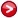# List of operators with four units of lepton number

In the paper "Renato M. Fonseca and Martin Hirsch, $\Delta L \ge 4$ processes, arXiv:1804.10545 [hep-ph]" we discuss the prospects of observing violation of lepton number in four or more units. There, some important non-renormalizable operators with Standard Model fields are mentioned. In the pdf file below, for completeness, we provide all such $\Delta L =4$ operators up to dimension 22. The fields are the left-handed Weyl fermions $u^{c}=\left(\overline{\boldsymbol{3}},\boldsymbol{1},-2/3\right)$, $d^{c}=\left(\overline{\boldsymbol{3}},\boldsymbol{1},1/3\right)$, $Q=\left(\boldsymbol{3},\boldsymbol{2},1/6\right)$, $e^{c}=\left(\boldsymbol{1},\boldsymbol{1},1\right)$, $L=\left(\boldsymbol{1},\boldsymbol{2},-1/2\right)$, and the scalar $H=\left(\boldsymbol{1},\boldsymbol{2},+1/2\right)$. We denote derivatives, which need to be covariant, with a $\partial$. Conjugation of fermions and scalars is denoted with bars and stars, respectively.

The pdf file with the list:Author
Renato Fonseca

Emails
renatofonseca@gmail.com
or
renatofonseca@ugr.es

Last updated
13 December 2021